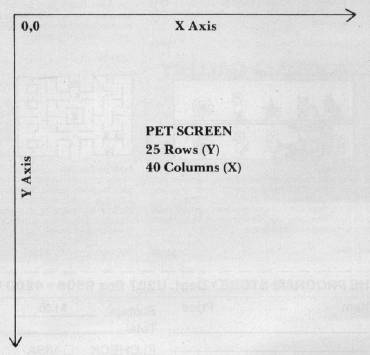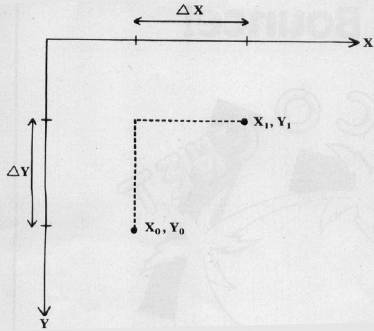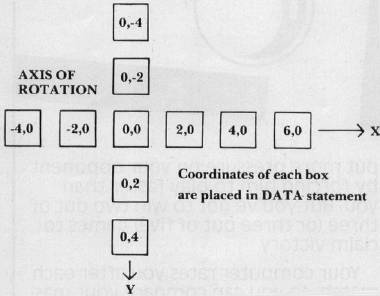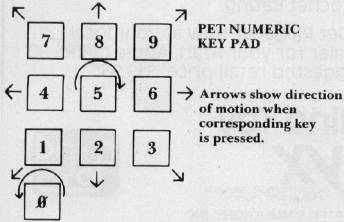`COMPUTE! ISSUE 26 / JULY 1982 / PAGE 68`

Screen Graphics

Ian Paull
Rochester, NY

How to move and rotate objects on screen. These ideas are adaptable to many computer graphics problems and can ease the task of creating games, simulations, etc. An Atari and Commodore version are presented as complete programs and the author describes the modifications necessary for other computers.

Many computer simulations and games use graphics to represent a mathematical event or model in the program. In a lunar lander program, for example, the lander position is first calculated by the model (taking operator input into account) and then displayed. Another simulation might calculate how a machine or robot should be responding to computer control, and then graphically display this information.

Programs of these general types can benefit from graphics routines which can illustrate an object at any location on a video screen (translation) and at any angle (rotation). The BASIC program presented here uses a 25 × 40 screen area to display a user-generated figure at any location and angle that the screen can represent.

The screen is treated as an X Y coordinate system, with the X axis running from left to right, the Y axis running from top to bottom, and the origin (0,0) located at the upper left corner. (See Figure 1.) The plotting routine is the main section which can be modified. Briefly, line 1060 clears the screen. Line 1080 computes the memory address to be POKEd, from the X and Y values computed elsewhere. The POKEd address is the screen position which will be turned on. Line 1090 insures that only points on the screen will be POKEd. This line may be deleted for slightly faster running time if you are sure to always remain on screen.

Lines 1070 to 1110 form a loop that plots all points in the figure to be displayed. For computers with continuous memory mapped display, you may need to change the values of SS (screen start address), SE (screen end address), RL (row length), and the character code for a completely lit cell (160 for the PET, line 1100). The PET can support greater resolution (50 × 80) with additional software, replacing the PLOT subroutine. (See references 1 and 2.)

Visible Errors

Note that due to the coarseness of the display, rotated lines will often appear warped. This is because, with only a limited number of points on the screen to choose from, the computer must pick points as close as it can to the spot where the point should go, often resulting in visible error. This program is thoroughly REMarked, so the user should have little difficulty changing or omitting statements to suit his particular needs or computer.

The subroutines actually doing the graphics manipulations begin at line 830 for rotation and 970 for translation. Since translation is simpler, let's look at it first.

In moving a point from one spot on a graph to another, the X and Y processing can be handled separately. (See Figure 2.) In travelling from point X0, Y0, to X1, Y1, the difference in X (shown as ΔX) is simply added to X0, and the difference in Y, a negative value in this example (shown as ΔY), is added to Y0. For this single point translation, then:

X1 = X0 + ΔX

Y1 = Y0 + ΔY

Subroutine 970 performs these additions for each translation of X and Y, for each point in the figure, and replaces the old X and Y values for each point with the new values. It also keeps a running total on the X and Y translation, to be used in the Rotation subroutine. Also, the Plot routine is called here to display the new, translated points.

The Rotation subroutine at line 830 is more complicated. The relationship between an existing point (X0, Y0) and the new computed point (X1, Y1) can be expressed as:

X1 = X0 cos (a) - Y0 sin (a)

Y1 = X0 cos (a) + Y0 cos (a)

where a is the angle of rotation. For details on the derivation of these relationships, see reference 3.

The Method Of Rotation

These equations describe rotation of a point about the origin. On initial entry of data for the pattern to be displayed, coordinates are chosen so the axis of rotation is at the origin. Then, if we later wish to rotate about this axis after the figure has been translated elsewhere on the screen, we must first translate the figure back to the origin, do the rotation, and then translate back to where the figure had been. Naturally, these intermediate steps are not displayed on the screen.

The pattern of points which makes up the displayed figure is in the DATA statement of line 230. The first number is an X value, next comes its associated Y value, and so on until all points are described. The sequence in which points are described here is the sequence with which they will be plotted. (See Figure 3.)

Before branching to the ROTATION subroutine, the following variables must be initialized:

NP = Number of points in displayed pattern.

IA = Incremental angle of rotation, in radians.

AX, AY = Absolute X and Y values that the axis of rotation has been translated from the origin.

XP (NP), YP (NP) = Arrays containing X Y coordinates.

Before branching to the Translation subroutine, you must initialize:

XT, YT = Value of X and Y translation.

NP, XP (NP), YP (NP) = As before.

Note that AX and AY are normally updated in the Translation subroutine and should be set to zero at the start of the program.

When entering this program, the user can, if desired, omit all REMarks, since there are no branches to REM statements. When running the program, the figure will first appear in the upper left of the screen, partially hidden by the screen edge and wrapped around the display. Pressing the numeric keys translates the figure, a lop-sided plus sign, according to the standard scheme of Figure 4. In addition, pressing the zero will rotate the pattern 15 degrees counter-clockwise, and pressing the five will rotate 15 degrees clockwise.

As with most BASIC programs which manipulate graphics, these routines work slowly with large numbers of points. The ten point example does one rotation in little more than one second. If the program must rotate quickly, either the incremental angle of rotation can be increased, or sections of this program can be rewritten in machine language.

References

1) High-Resolution Plotting for the PET, J. Sherburne, Micro No. 10, March 1979, Machine language routine.

2) Workbook 3, Total Information Services, Basic routine for hi-res. plotting, PET.

3) The Mathematics of Computer Graphics, J. Posdamer, Byte Vol. 3, No. 9, Sept. 1978.

 Notes For Atari Users The Atari version of this routine closely parallels the PET version. Instead of screen POKEs, ordinary X,Y PLOTting is used, and instead of using the numeric keypad to generate the values for the translation routine, this program uses a joystick to move (translate) the figure around the screen. The less-than "<" and greater-than ">" keys are used to rotate the figure. Because of the general nature of this program, it can be run in Applesoft BASIC with only minor changes, such as changing GRAPHICS 3 + 16 to GR ; COLOR 1 to COLOR = 1, TRAP to ONERR GOTO, GET # 1, A to GET A\$ : A = ASC(A\$), etc., and using the keyboard, paddles, or a joystick to get the values for XT and YT (1, 0, or -1).
 Other Machines: If your BASIC supports X, Y PLOTting, use the Atari version as a guide, otherwise modify variables SS, SE, and RL in the PET/CBM version if you have a memory-mapped text display. (See the article for details.)
Figure 1.Figure 2.Figure 3.Figure 4.Program 1. Commodore Microsoft Version

```100 REM 2-D GRAPHICS SUBROUTINE DEMO
110 REM BY IAN PAULL
120 PRINT "{CLEAR}" : REM CLEAR SCREEN
130 DIM XP(10), YP(10) : REM ENLARGE FOR MORE ~ POINTS
140 REM INITIALIZE
150 POKE 59468, 12 : REM PUTS PET IN STANDARD ~ CHARACTER MODE
160 NP = 10 : SS = 32768 : SE = 33767 : RL = 40 : AX = 0 : AY = 0
170 REM NP = NUMBER OF POINTS; SS = SCREEN START ADDRESS ; SE = SCREEN END ADDRESS
180 REM RL = ROW LENGTH; AX, AY = ACCUMULATED X ~ AND Y VALUES (NET XLATION SO FAR)
190 FOR KZ = 1 TO NP : REM READ FIGURE COORDS
210 NEXT KZ
220 REM DATA BELOW ARE FIGURE COORDS. FORMAT FOR EACH POINT IS X, Y
230 DATA - 4, 0, -2, 0, 0, 0, 2, 0, 4, 0, 6, 0, 0, 4, 0, 2, 0, -2, 0, -4
240 GOSUB 1060 : REM PLOT
250 GETA\$ : IFA\$-"" THEN 250 : REM LOOPS UNTIL AN INPUT
260 A = VAL(A\$) + 1 : REM CONVERTS STRING INPUT TO NUMERIC VALUE
270 ON A GOTO 290, 370, 420, 470, 520, 330, 570, 620, 670, 720
280 REM CCW ROTATE, FIXED ANGLE 15 DEG
290 IA = -(15/360)* (2*) : REM_ = PI
300 GOSUB 780 : REM ROTATE ROUTINE
310 GOTO 250
320 REM CW ROTATE, FIXED ANGLE 15 DEG
330 IA = (15/360)*(2*) : REM_=PI
340 GOSUB 780 : REM ROTATE ROUTINE
350 GOTO 250
360 REM XLATE DOWN AND LEFT
370 XT = -1
380 YT = 1
390 GOSUB 970 : REM XLATE ROUTINE
400 GOTO 250
410 REM XLATE DOWN
420 XT = 0
430 YT = 1
440 GOSUB 970 : REM XLATE ROUTINE
450 GOTO 250
460 REM XLATE DOWN AND RIGHT
470 XT = 1
480 YT = 1
490 GOSUB 970 : REM XLATE ROUTINE
500 GOTO 250
510 REM XLATE TO LEFT
520 XT = -1
530 YT = 0
540 GOSUB 970
550 GOTO 250
560 REM XLATE TO RIGHT
570 XT = 1
580 YT = 0
590 GOSUB 970
600 GOTO 250
610 REM XLATE UP AND LEFT
620 XT = -1
630 YT = -1
640 GOSUB 970
650 GOTO 250
660 REM XLATE UP
670 XT=0680 YT = -1
690 GOSUB 970
700 GOTO 250
710 REM XLATE UP AND TO RIGHT
720 XT = 1
730 YT = -1
740 GOSUB 970
750 GOTO 250
760 REM ROTATE CONTROL
770 REM FIRST, XLATE TO ORIGIN
780 FOR KZ = 1 TO NP
790 XP (KZ) = XP(XZ) - AX
800 YP (KZ) = YP(KZ) - AY
810 NEXT KZ
820 REM DO ROTATION ABOUT 0, 0
830 SA = SIN (IA) : CA = COS(IA)
840 FOR KZ = 1 TO NP
850 KN = XP(KZ)
860 XP(KZ) = KN * CA - YP(KZ) * SA
870 YP(KZ) = KN * SA + YP(KZ) * CA
880 NEXT KZ
890 REM XLATE BACK
900 FOR KZ = 1 TO NP
910 XP(KZ) = YP(KZ) + AX
920 YP(KZ) = YP(KZ) + AY
930 NEXT KZ
940 GOSUB 1060 : REM PLOT
950 RETURN
960 REM XLATE
970 AX = AX + XT : REM KEEP TRACK OF ABS X
980 AY = AY + YT : REM KEEP TRACK OF ABS Y
990 FOR KZ = 1 TO NP : REM SHIFT X AND Y
1000 XP(KZ) = XP(KZ) + XT
1010 YP(KZ) = YP(KZ) + YT
1020 NEXT KZ
1030 GOSUB 1060 : REM PLOT
1040 RETURN
1050 REM PLOT
1060 PRINT " {CLEAR} " REM CLR SCREEN
1070 FOR KZ = 1 TO NP : REM FIGURE ADDRESSES TO ~ BE POKED
1080 LOC = (SS + RL * INT(YP(KZ) + .5) + INT(XP(KZ) + .5))
1090 IF LOC<SS OR LOC>SE THEN NEXT KZ : RETURN : REM DON'T POKE OFF SCREEN
1100 POKE LOC, 160 : REM LIGHT CHARACTER CELL AT SCREEN LOCATION ‘LOC’
1110 NEXT KZ
1120 RETURN
1130 END
```

Program 2. Atari Version

```110 OPEN #1, 4, 0, "K"
120 GRAPHICS 3 + 16
130 DIM XP(10), YP(10) : REM ENLARGE DIMENSION FOR MORE POINTS
140 REM INITIALIZE
160 AX = 0 : AY = 0 : NP = 10 : PI = 3.1415927
170 REM NP = NUMBER OF POINTS
190 FOR KZ = 1 TO NP
200 READ T : XP(KZ) = T : READ T :  YP(KZ) =  T
210 NEXT KZ
220 REM DATA BELOW ARE FIGURE COORDS.
225 REM FORM FOR EACH POINT IS X, Y
230 DATA -4, 0, -2, 0, 0, 0, 2, 0, 4, 0, 6, 0, 0, 4, 0, 2, 0, -2, 0, -4
240 GOSUB 1060 : REM PLOT
250 IF PEEK(764)< 255 THEN 300
260 ST = STICK(0) : IF ST = 15 THEN 250
270 XT = -(ST>8 AND ST<12) + (ST>4 AND ST<8)

280 YT = (ST = 9 OR ST = 5 OR ST = 13) - (ST = 10 OR ST = 14 OR ST = 6)
290 GOSUB 970 : REM XLATE ROUTINE
295 GOTO 250
300 GET #1, A
310 IF A<>60 AND A<>62 THEN 250
320 IA = (-(A = 60) + (A = 62)) * (15/360) * (2 * PI)
330 GOSUB 780 : REM ROTATE ROUTINE
340 GOTO 250
760 REM ROTATE CONTROL
770 REM FIRST, XLATE TO ORIGIN
780 FOR KZ = 1 TO NP
790 XP(KZ) = XP(K2) - AX
800 YP(KZ) = YP(KZ) - AY
810 NEXT KZ
820 REM DO ROTATION ABOUT 0, 0
830 SA = SIN(IA) : CA = COS(IA)
840 FOR KZ = 1 TO NP
850 KN = XP(KZ)
860 XP(KZ) = KN * CA - YP(KZ) * SA
870 YP(KZ) = KN * SA + YP(KZ) * CA
880 NEXT KZ
890 REM XLATE BACK
900 FOR KZ = 1 TO NP
910 XP(KZ) = XP(KZ) + AX
920 YP(KZ) = YP(KZ) + AY
930 NEXT KZ
940 GOSUB 1060 : REM PLOT
950 RETURN
960 REM XLATE
970 AX = AX + XT : REM KEEP TRACK OF ABS X
980 AY = AY + YT : REM KEEP TRACK OF ABS Y
990 FOR KZ = 1 TO NP : REM SHIFT X AND Y
1000 XP(KZ) = XP(KZ) + XT
1010 YP(KZ) = YP(KZ) + YT
1020 NEXT KZ
1030 GOSUB 1060 : REM PLOT
1040 RETURN
1050 REM PLOT
1060 GRAPHICS 3 + 16 : SETCOLOR 0, 0, 10 : COLOR 1
1070 FOR KZ = 1 TO NP
1080 TRAP 1090
1085 PLOT XP(KZ), YP(KZ) : TRAP 40000
1090 NEXT KZ
1100 RETURN
1130 END
```